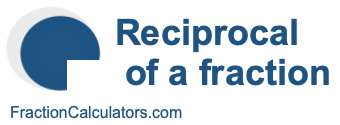Reciprocal of 4/6What is the reciprocal of 4/6? Here we will define reciprocal of 4/6, and show you how to calculate the reciprocal of 4/6 in fraction form and decimal form.

The reciprocal of 4/6 is a fraction or a number that when multiplied by 4/6 is equal to 1. To get the reciprocal of 4/6 based on that definition, we can make the following equation where "R" is the reciprocal of 4/6.

(4/6) × R = 1

When we solve for R, we get the answer to the reciprocal of 4/6 as a fraction as follows:

(4/6) × R = 1
R = 6/4
Reciprocal of 4/6 = 6/4

You can check that the answer is correct by confirming that 4/6 times the reciprocal of 4/6 is equal to 1, like this:

4/6 × 6/4 = 1

To find the decimal answer to the reciprocal of 4/6, you divide the numerator of the reciprocal by the demoninator of the reciprocal, like this:

6 ÷ 4 = 1.5
Reciprocal of 4/6 = 1.5

Tip: As you may have inferred from our tutorial above, you can quickly get the reciprocal of any fraction, such as 4/6, by switching the numerator and the denominator.

Reciprocal of a fraction
Do you need the reciprocal of another fraction? No problem! Please enter another fraction below.

Reciprocal of /

Reciprocal of 4/7
Here is the next fraction on our list that we have calculated the reciprocal of.